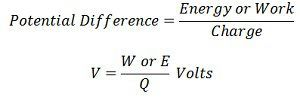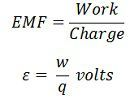# Difference Between Electromotive Force & Potential Difference

The potential difference and electromotive force (emf) both is the form of energy. One of the major difference between the emf and potential difference is that the emf is caused by converting the other form of energy into electrical energy whereas in potential difference the electrical energy is converted into other forms of energy. Some other differences between them are explained below in the form of the comparison chart.

## Comparison Chart

Basis For ComparisonElectromotive ForcePotential Difference
DefinitionIt is the amount of energy supply to one coulomb of charge.The amount of energy used by one coulomb of charge in moving from one point to another.
UnitVoltVolt
SymbolεV
Source
Dynamo or BatteryBattery
Resistance Independent from the resistance of the circuit Proportional to the resistance of the circuit.
Current It transmits current throughout the circuit. It transmits current between any two points.
Magnitude Greater than the potential difference between any two pointsAlways less than the maximum value of emf when the
battery is fully charged.
Variation It remains constantDoes not remain constant.
Relation CauseEffect
No current Not Zero Zero
VoltageIt is the maximum voltage that the battery can transfer It is less than the maximum voltage that cell can deliver.
Field Causes in magnetic, gravitational and electric field. Induces only in electric field
Energy Gain energy Loss energy
Measuring Instrument
EMF Meter Voltmeter

## Definition of Potential Differences

The potential difference is defined as the amount of energy used by one coulomb of charge in moving from one point to the other. It is measured in volts and represented by the symbol  V. The potential difference is measured by the Voltmeter.The potential difference between the two point charges is expressed by the formula shown below.Example – Consider a circuit shown in the figure below.A 12 volt supply applies across the resistance of the circuit. The potential difference between any two points, says A and B, is the energy used by one coulomb of charge in moving from one point (A) to the other (B). Thus, the potential difference between point A and B  is 7 volts.

## Definition of Electromotive force

The electromotive force is the total voltage induce by the source. In other words, it is the amount of energy supplied by the source to each coulomb of charge. It is measured in volts and represented by the symbol ε (epsilon).The emf is the maximum voltage that can be attained by the circuit. Naturally, it is generated when the fluctuation occurs in the magnetic field. The emf is expressed by the formula shown belowThe electromotive force is the type of energy which forces a unit positive charge to move from the positive to the negative terminal of the source. It separates the two charges from each other.

Example – Consider a circuit shown in the figure below.A battery has an EMF of 12V ; it means that the battery supplies 12 joules of energy to each coulomb of charge. The charge is travel from the positive terminal to negative terminal through an external circuit; it gives a whole of the energy originally supplied by the battery.

## Key Differences between Electromotive Force and Potential Difference.

1. The electromotive force is the measure of energy that it gives to each coulomb of charge, whereas the potential difference is the amount of energy used by the one coulomb of charge.
2. The electromotive force is represented by the symbol ε whereas the symbol V represents the potential difference.
3. The electromotive force does not depend on the internal resistance of the circuit whereas the potential difference is directly proportional to the resistance of the circuit.
4. The electromotive force transfers the energy in the whole of the circuit. The potential difference is the measure of energy between any two points on the circuit.
5. The magnitude of the electromotive force is always greater than the potential difference when the circuit is unchanged but when the circuit is fully charged the magnitude of the potential difference is equal to the emf of the circuit.
6. The magnitude of emf has always remained constant, whereas the magnitude of the potential difference varies.
7. The electromotive force is the cause of the potential difference, whereas the potential difference is the effect of the potential difference.
8. The emf force exists in the circuit even when the current does not flow in the circuit whereas the potential difference does not exist in the circuit when the magnitude of current remain zero.
9. The  EMF is the maximum voltage that the battery can deliver whereas the magnitude of the potential difference is always less than the maximum possible value of emf.
10. The emf force gains the electrical energy in the circuit whereas the potential difference loss the electrical energy in the circuit.
11. The electromotive force is induced in the electric, magnetic and gravitational field, whereas the potential difference is generated only in an electric field.
12. The emf meter is used for measuring the electromotive force, whereas the voltmeter is used for measuring the potential difference.

### Point to Remember

At first sight, the name emf implies that it is a force that causes the electrons (charged particles, i.e., current) to flow through the circuit. In fact, it is not a force, but it is energy supplied by some active source such as a battery to one coulomb of charge.

### 11 thoughts on “Difference Between Electromotive Force & Potential Difference”

1. Kenneth Anierobi

I really want to say a very thank you ❤ 😊 I believe this will make things easier for us I really appreciate . God bless you Amen

2. Rogue Abdulrafiu

Thanks a lot, it helped me in understanding the difference between electromotive force and potential difference.
Keep up the good work. God bless…